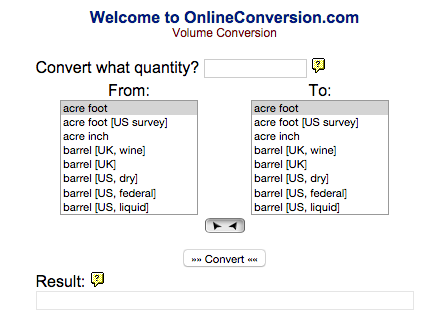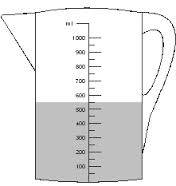# How many milliliters are in a liter?

1,000, of course!

1 Person thanked the writer.

There are 1000 millilitres in a litre.

The prefix "milli" is actually derived from the Latin for 1000, so the term denotes that there are 1000 millilitres within a litre.

You can find conversion tools for working out volume problems at Onlineconversion.com - just click on the image below.Millilitres and litres are the most common unit of measurement used to measure volumes of substances such as liquids, and form part of the "metric system".

Some examples of everyday liquids that we use millilitres and litres for are:

• Water
• Milk
• Petrol
• Other drinks
• Blood
• Paint

However the following substances are also referred to using millilitres and litres:

• Liquid oxygen
• Liquid metal

One litre is equal to the volume of a cube that is made up of sides with 10cm.

The shortening of a litre is 'l' and is always in lower case. Only symbols that are the shortening of a persons name use a capital letter.The first introduction of a litre was in 1795, and was just used just in France at this time. It was not until 1879, that the symbol was used elsewhere.

The shortening of a millilitre is 'ml'. It is also sometimes known as a cubic centimetre. A millilitre is a smaller unit of measurement to a litre so is used to give more precise results.

The best way to calculate the amount of liquid you have, is by using a measuring jug. These are usually made of clear plastic or glass, so that you can see the amount of liquid that is inside of the jug. You will find the measurements running up the side of the glass so you can compare the liquid inside to the points on the glass.

On some measuring jugs, points will be marked for quarter, half and three quarters of a litre, which can be very helpful.

thanked the writer.